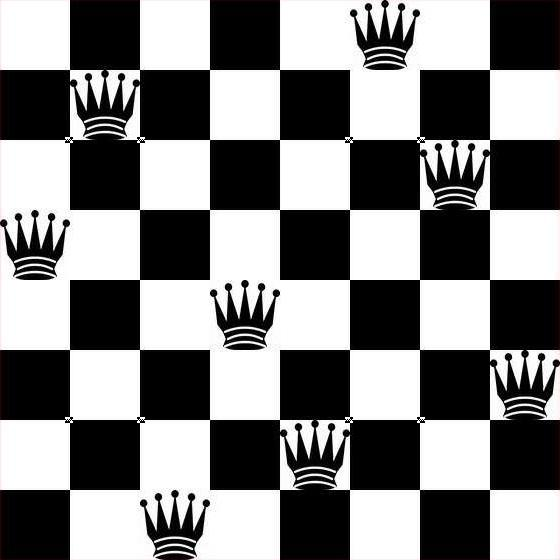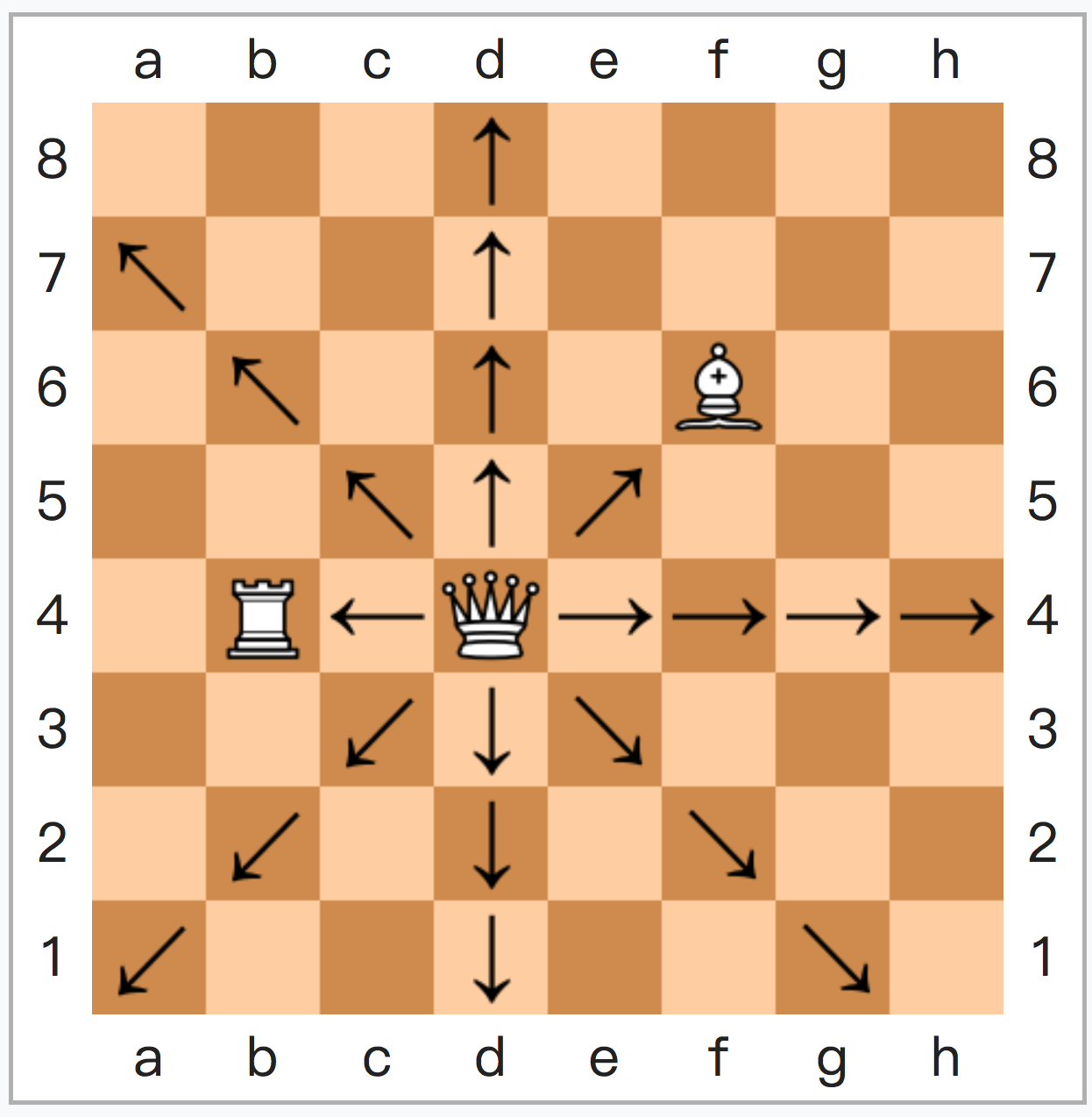方丈記 – 逝水轻尘（鴨長明）【原文】

ゆく川のながれは绝えずして、しかも、本の水にあらず。よどみに浮ぶうたかたは、かつ消えかつ结びて久しくとゞまりたる例なし。世中にある、人と栖と、またかくの如し。

たましきの都のうちに、棟を並べ、甍を争へる、高き、いやしき、人の住ひは、世々を經て盡きせぬものなれど、これをまことかと尋（ぬ）れば、昔しありし家は稀なり。或は去年焼けて今年作れり。或は大家滅びて小家となる。住む人もこれに同じ。所も変らず、人も多かれど、いにしへ见し人は、二三十人が中に、わづかにひとりふたりなり。朝に死に、ゆふべに生るゝならひ、たゞ水の泡にぞ似たりける。不知、生れ死（ぬ）る人、何方より来たりて、何方へか去る。また不知、假の宿り、谁が為にか心を恼まし、何によりてか目を喜ばしむる。その、主と栖と、无常を争ふさま、いはゞ朝颜の露にことならず。或は露おちて花のこれり。残るといへども朝日に枯れぬ。或は花はしぼみて露なほ消えず。消えずといへどもゆふべを待つことなし。

【譯文】

N个女人的老死不相往来——n皇后问题n皇后问题，较早为八皇后问题，出现于1848年。
Max Bezzel讨论的是“在国际象棋上出现8个后时，（八皇后问题的其中一个解）

（一方全升变，一方被吃过路兵一次，加上初始两个）

——直到图论和函数式编程思想出现，

' n queens cast by go basic
[loop]
input "How many queens (N>=4)";n
if n < 4 then
print "Must be greater than 4"
goto [loop]
end if

dim plot$(100,100) dim q(n+20) dim e(n+20) dim o(n+20) r=n mod 6 if r<>2 and r<>3 then gosub [samp] goto [shoBoard] end if for i=1 to int(n/2) e(i) = 2 * i next for i=1 to int((n/2)+.5) o(i) = 2 *i-1 next if r = 2 then gosub [edt2] if r = 3 then gosub [edt3] s = 1 for i=1 to n if e(i)>0 then q(s) = e(i) s = s+1 end if next for i=1 to n if o(i) > 0 then q(s) = o(i) s = s + 1 end if next ' print board [shoBoard] cls for i = 1 to n plot$(i,26-q(i)) = "*"
plot$(i,24-n) = chr$(96+i)
plot$(n+1,26-i) = str$(i)
next i
for ii = 1 to 100
for jj = 1 to 100
print left$(plot$(jj,ii)+" ",1);
next jj
print
next ii
end

' the simple case
[samp]
p = 1
for i = 1 to n
if i mod 2=0 then
q(p) = i
p    = p + 1
end if
next i
for i = 1 to n
if i mod 2 then
q(p) = i
p    = p + 1
end if
next
return
' edit list when remainder is 2
[edt2]
for i=1 to n
if o(i) = 3 then
o(i) = 1
else
if o(i)=1 then o(i) = 3
end if
if o(i) = 5 then
o(i)= o(i) -1
else
if o(i) = 0 then
o(i) = 5
return
end if
end if
next

' edit list when remainder is 3
[edt3]
for i = 1 to n
if e(i) = 2 then
e(i)  = e(i)-1
else
if e(i) = 0 then
e(i) = 2
goto [more]
end if
end if
next i
' edit list some more
[more]
for i = 1 to n
if (o(i)=1 or o(i)=3) then
o(i) = o(i)-1
else
if o(i) = 0 then
o(i)   = 1
o(i+1) = 3
return
end if
end if
next

# Brute force permutations for 8 queens by R

safe <- function(p) {
n <- length(p)
for (i in seq(1, n - 1)) {
for (j in seq(i + 1, n)) {
if (abs(p[j] - p[i]) == abs(j - i)) return(F)
}
}
return(T)
}

queens <- function(n) {
p <- 1:n
k <- 0
while (!is.null(p)) {
if(safe(p)) {
cat(p, "\n")
k <- k + 1
}
p <- next.perm(p)
}
return(k)
}

queens(8)

——只会利用工具的人，终究会被工具抛弃。

N皇后问题中，穷举法的复杂度为(n!)，1 N皇后于NxN棋盘上，若老死不相往来，则绝不在同一行列。
2 攻击范围有重叠时，绝非边与边重叠，只可相交，且相交仅会出现一次。

（计算第5横行时，下子状态保留为 0001 0000，

#include <iostream>

// total:记录解；finishline:确认的行
int total = 0, finishline = 1;

// row:竖直攻击; ls:斜杠攻击; rs:反斜杠攻击
void trying(long row, long ls, long rs)
{
if (row != finishline) // 开始新行
{
long position = finishline & ~(row | ls | rs);
while(position) // 若行间无位可放...
{
long pointline = position & -position;
position -= pointline;
trying(row + pointline, (ls + pointline) << 1, (rs + pointline) >> 1);
}
}
else
{
total++;
}
}

using namespace std;

int main(int argc, char *argv[]) {
int grid = 8; // 修改grid的话，1～31皇后都能解w

finishline = (finishline << grid) - 1;

trying(0, 0, 0);

cout << total << endl;

return 0;

}

#include <iostream>
int total = 0, finishline = 1;
void trying(long row, long ls, long rs){
if (row != finishline) { long position = finishline & ~(row | ls | rs);
while(position) { long pointline = position & -position; position -= pointline; trying(row + pointline, (ls + pointline) << 1, (rs + pointline) >> 1); }} else {total++;}}
using namespace std;
int main(int argc, char *argv[]) {
int grid = 8; // 修改grid的话，1～31皇后都能解w
finishline = (finishline << grid) - 1; trying(0, 0, 0); cout << total << endl;
return 0;}

// 此处需使用八皇后算法，但忽略计算过程，仅使用答案节省时间。
// 八皇后解枚举数据头在******
void 8queensAnswers (0, 0, 0, 0)
//在里面做一个调用92个答案的接口，传参出去给大结构体
//保存各个答案类似于[91, 0, 4, 7, 5, 2, 6, 1, 3]这样
//或者n皇后的话[0,1][1,0][2,0][3,2][4,10][5,40]...这样

N皇后问题——记录下那些老死不相往来的N个女人的位置吧

(Syntax Highlight Testing#include &lt;iostream&gt;

using namespace std;

//此处开始搞事情

int main(int argc, char *argv[]) {

//此处开始搞事情

cout &lt;&lt; "此处开始练傻" &lt;&lt; endl;
return 0;
}

【翻译】Viva La Vida 我心不息 – ColdPlayI used to rule the world

Seas would rise when I gave the word

Now in the morning I sleep alone

Sweep the streets I used to own

I used to roll the dice

Feel the fear in my enemy’s eyes

Listen as the crowd would sing:

“Now the old king is dead
“先王老矣！”

Long live the king ”
“吾王万代！”

One minute I held the key

Next the walls were closed on me

And I discovered that my castles stand

Upon pillars of salt’ pillars of sand

I hear Jerusalem bells a ringing

Roman Cavalry choirs are singing

Be my mirror my sword and shield

My missionaries in a foreign field

For some reason I can’t explain

Once you gone there was never’

Never an honest word

That was when I ruled the world

It was the wicked and wild wind

Blew down the doors to let me in

Shattered windows and the sound of drums

People couldn’t believe what I’d become

Revolutionaries wait

For my head on a silver plate

Just a puppet on a lonely string

Oh who would ever wanna be king

I hear Jerusalem bells a ringing

Roman Cavalry choirs are singing

Be my mirror my sword and shield

My missionaries in a foreign field

For some reason I can’t explain

I know Saint Peter won’t call my name

Never an honest word

That was when I ruled the world

(Ohhhhh Ohhh Ohhh)

I hear Jerusalem bells a ringing

Roman Cavalry choirs are singing

Be my mirror my sword and shield

My missionaries in a foreign field

For some reason I can’t explain

I know Saint Peter won’t call my name

Never an honest word

That was when I ruled the world